# Forces Motion Test 1 Review A push or

• Slides: 53
Download presentation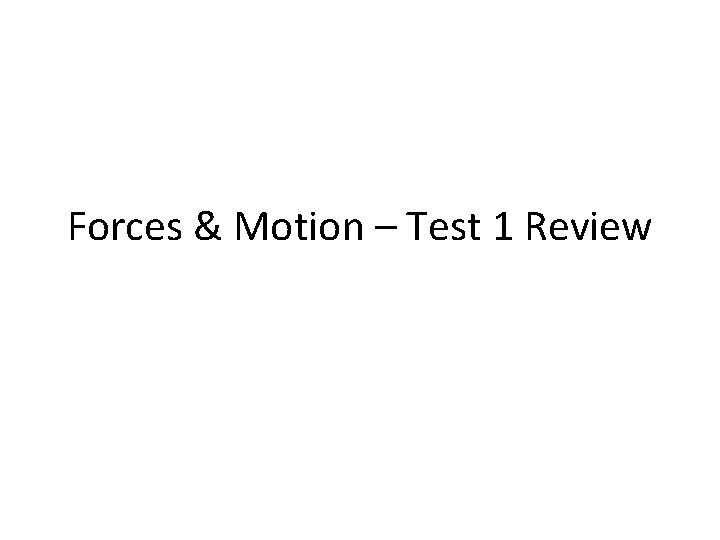Forces & Motion – Test 1 Review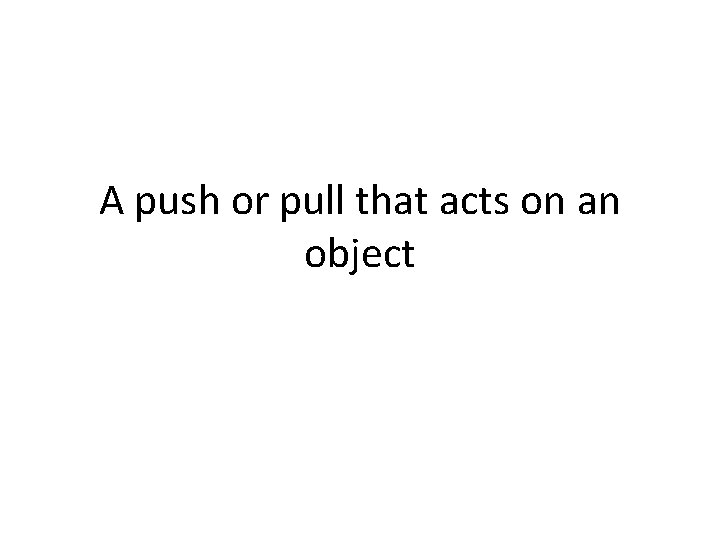A push or pull that acts on an object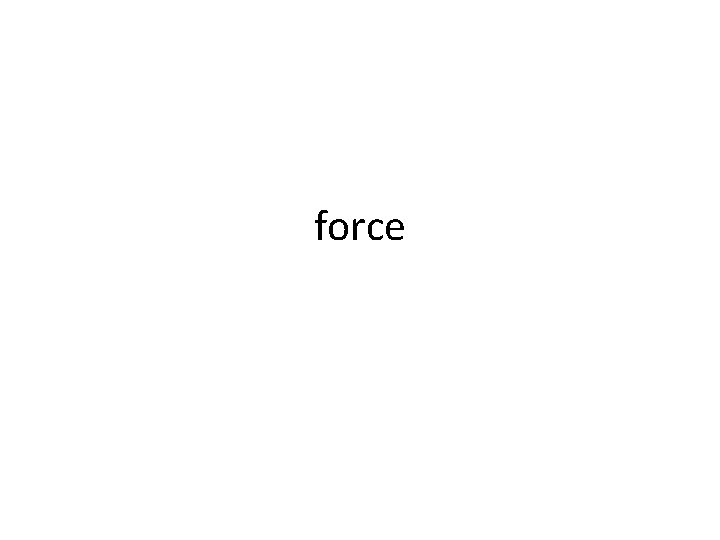forceA change in a moving object’s speed or direction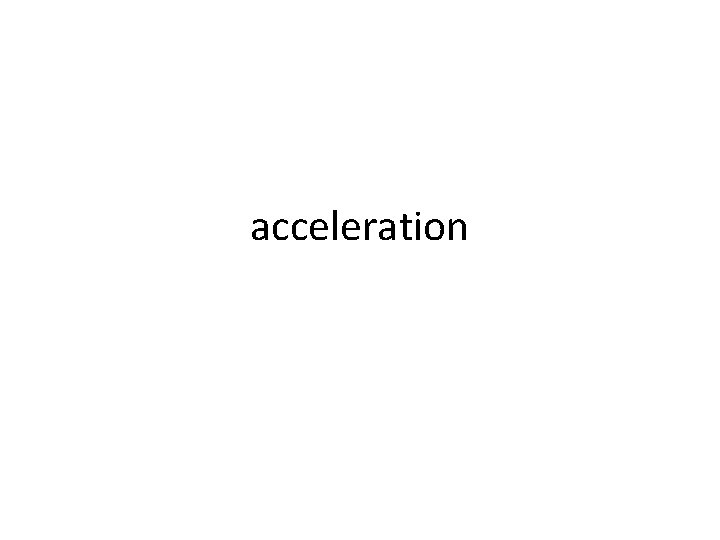accelerationA measure that does not change when an object’s location changesReference point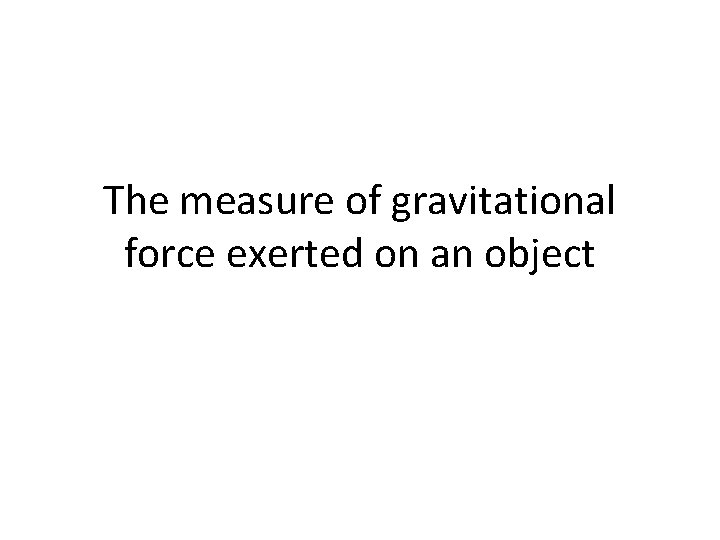The measure of gravitational force exerted on an objectweight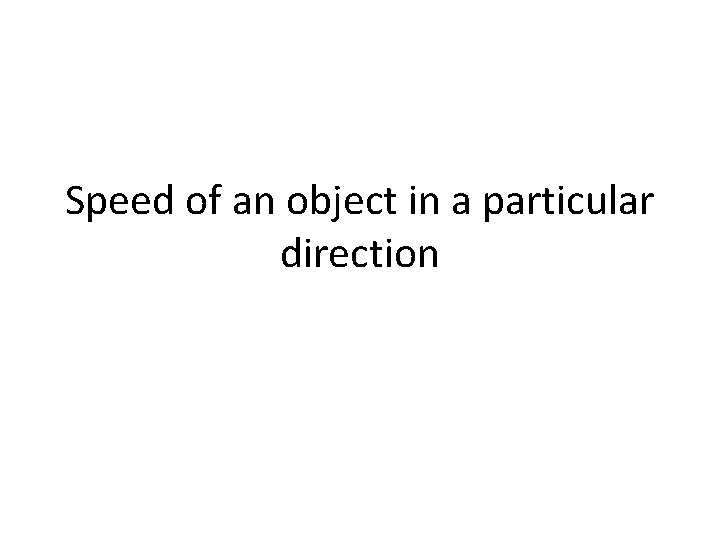Speed of an object in a particular directionvelocity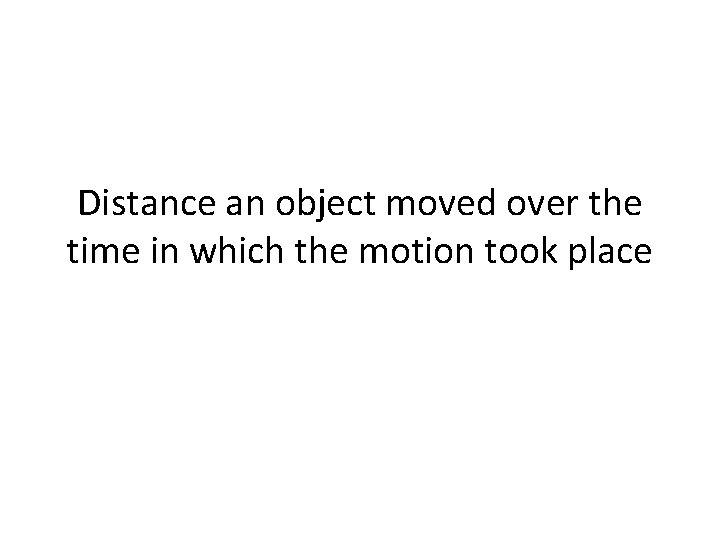Distance an object moved over the time in which the motion took placespeedThe SI unit force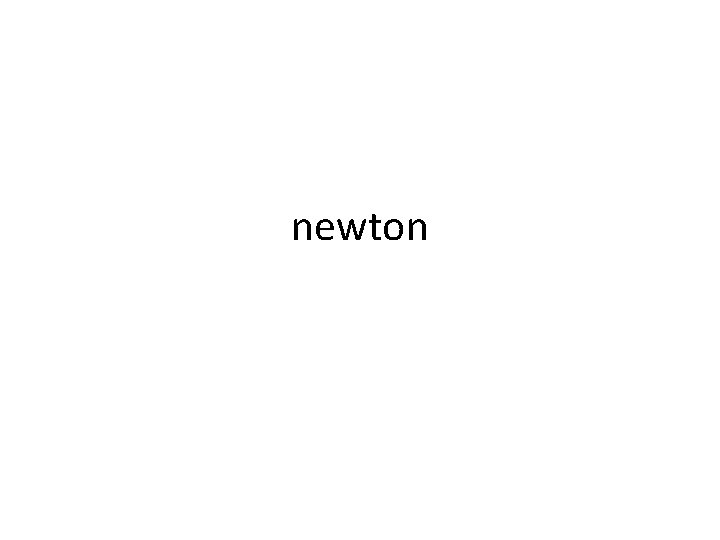newtonThe combination of all forces acting on an object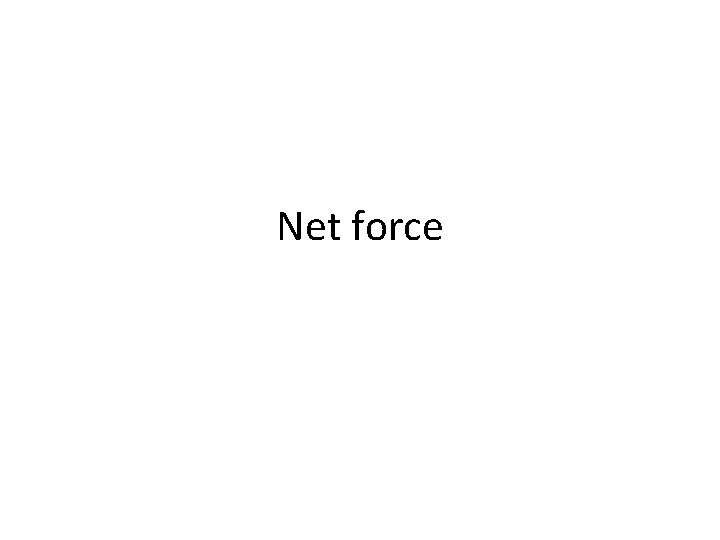Net forceTo calculate an object’s acceleration, what formula would you use?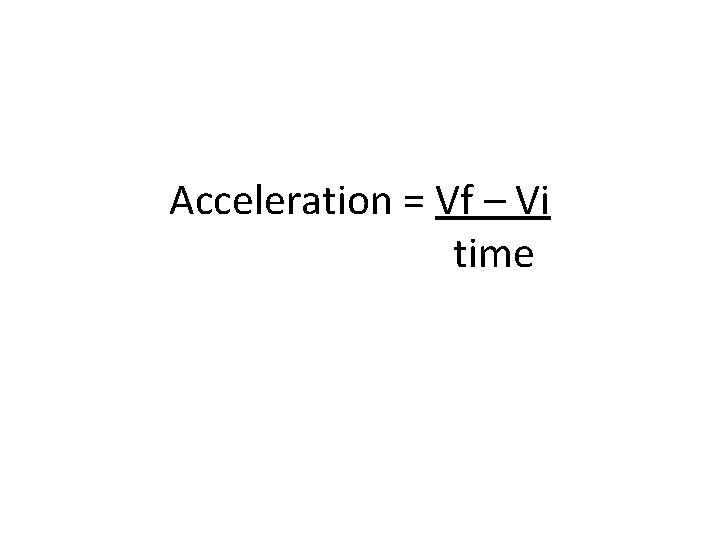Acceleration = Vf – Vi time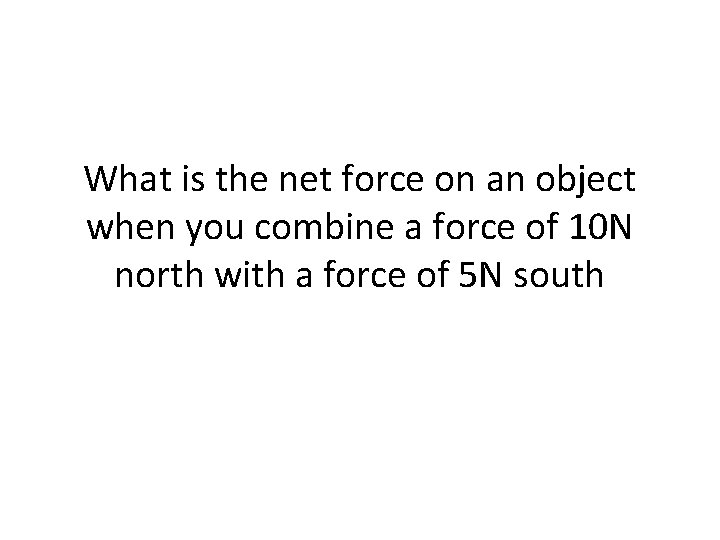What is the net force on an object when you combine a force of 10 N north with a force of 5 N south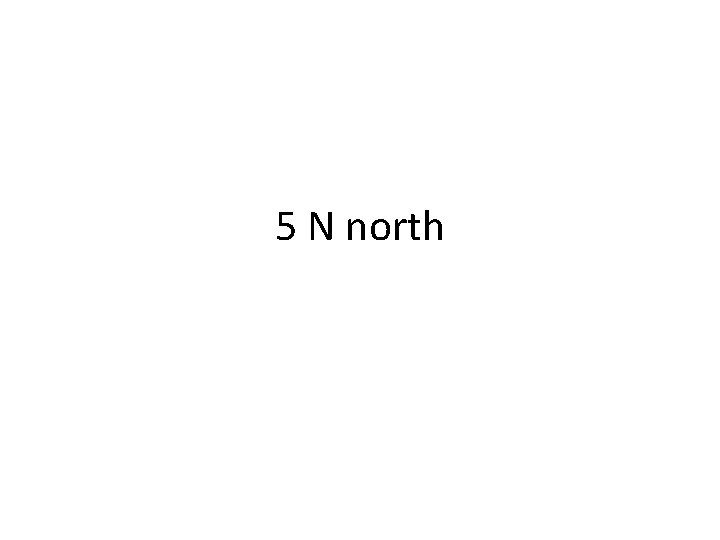5 N northDistance timespeed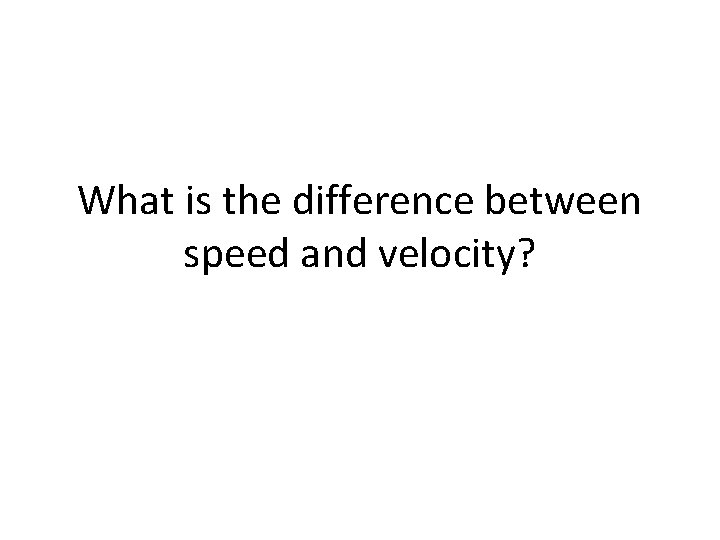What is the difference between speed and velocity?Velocity is in a certain direction, speed is not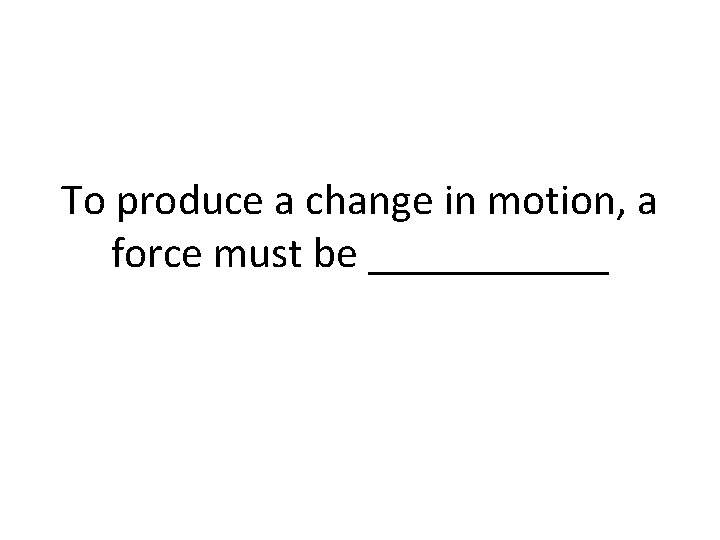To produce a change in motion, a force must be ______unbalanced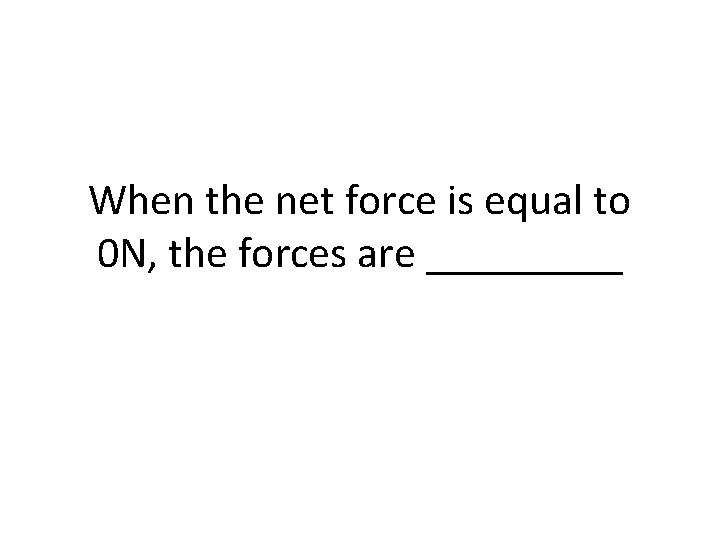When the net force is equal to 0 N, the forces are _____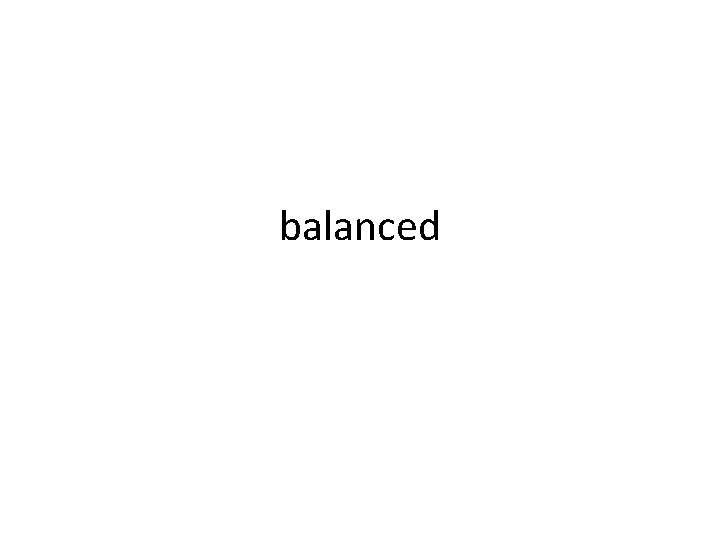balanced______ forces always cause a change in speed, direction, or bothunbalancedDoes a force applied always cause motion?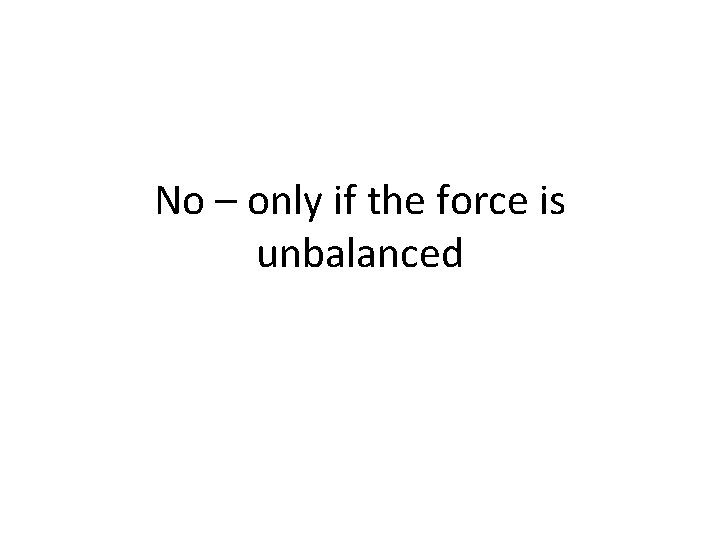No – only if the force is unbalanced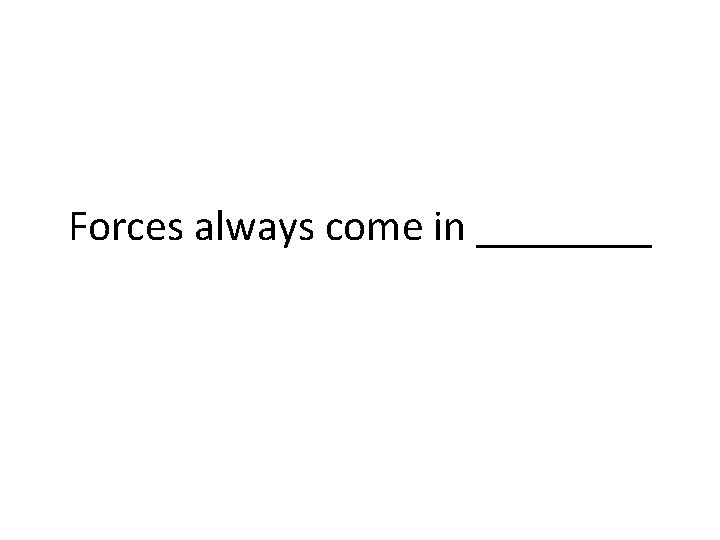Forces always come in ____pairs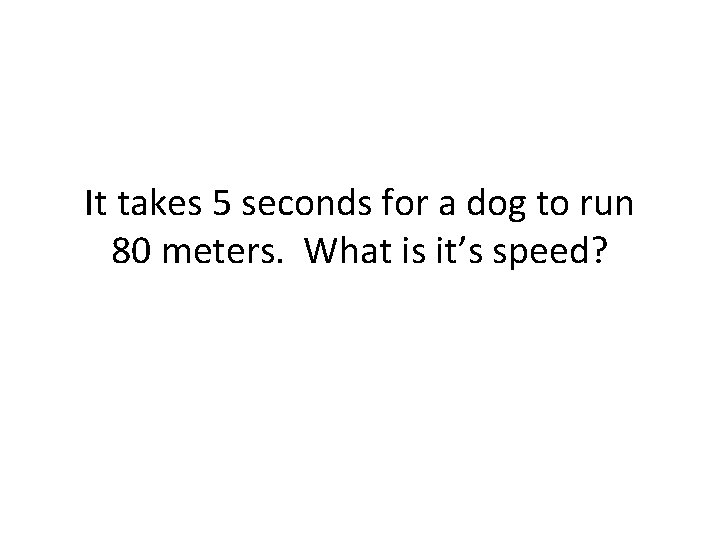It takes 5 seconds for a dog to run 80 meters. What is it’s speed?16 m/sA pig runs 84 meters in 7 seconds. What is it’s speed?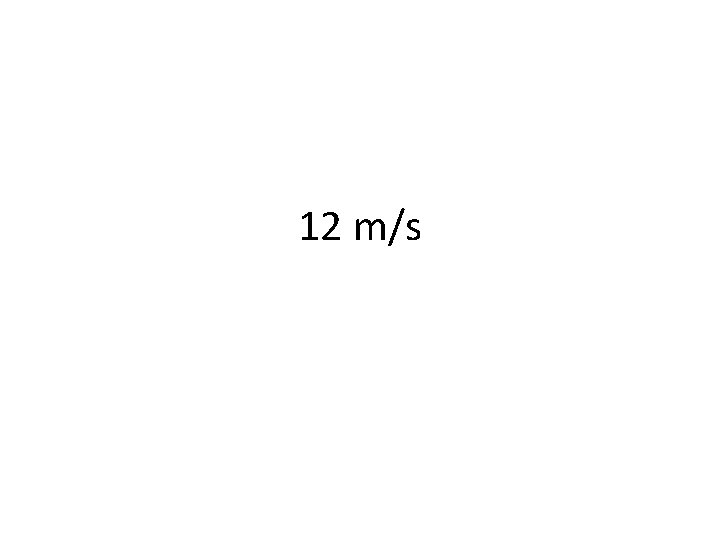12 m/sA track star runs the 100 meter dash in 10 seconds. What is his speed?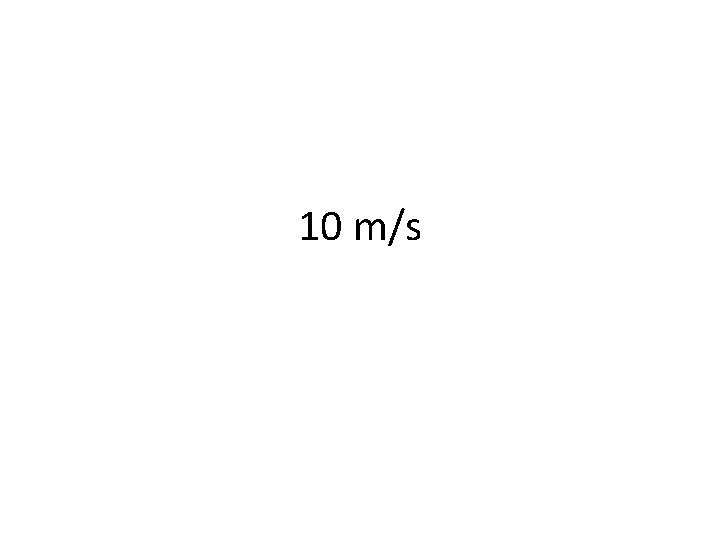10 m/s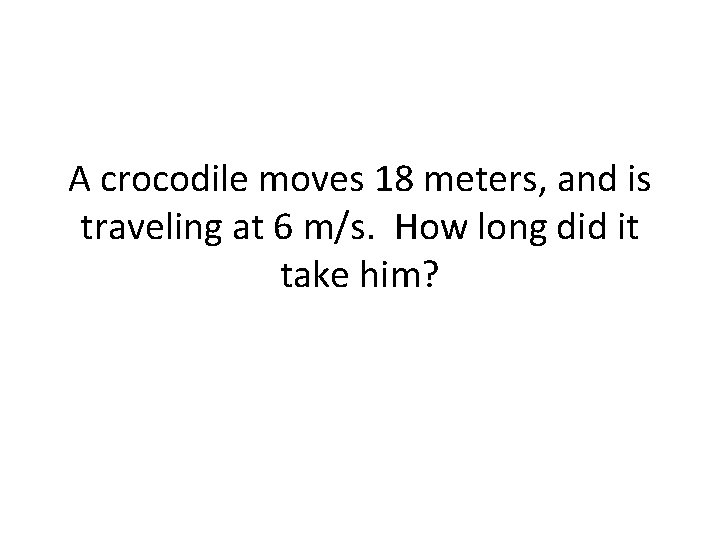A crocodile moves 18 meters, and is traveling at 6 m/s. How long did it take him?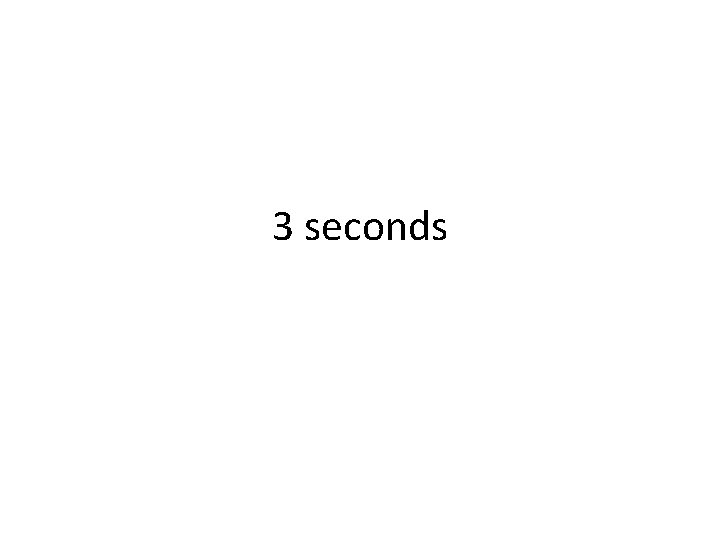3 secondsA plane traveled for 2. 5 hours at a velocity of 1200 km/hr. What distance did it travel?3000 kmA plane taking off reaches it’s takeoff velocity of 75 m/s after 5 seconds. What is it’s acceleration?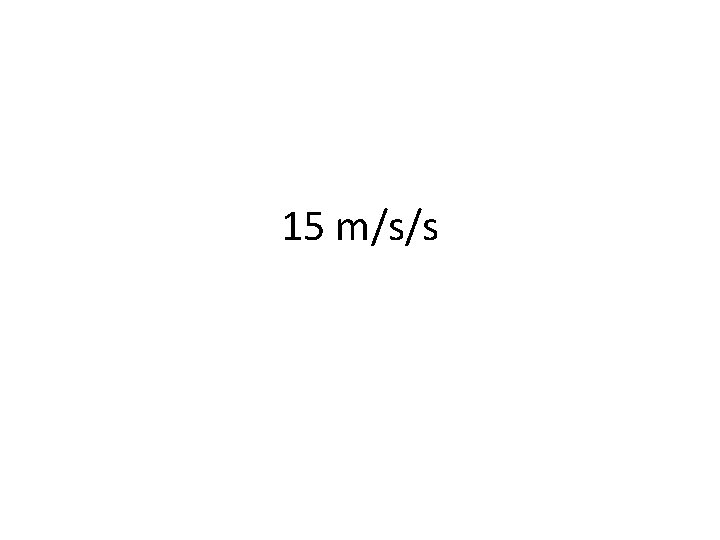15 m/s/sA ball rolling down a hill accelerates from 3 m/s to 34. 5 m/s in 9 seconds. What is it’s acceleration?3. 5 m/s/s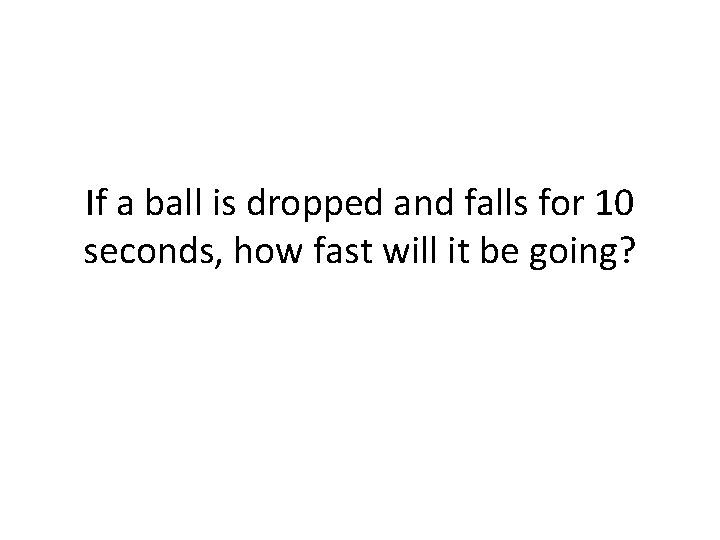If a ball is dropped and falls for 10 seconds, how fast will it be going?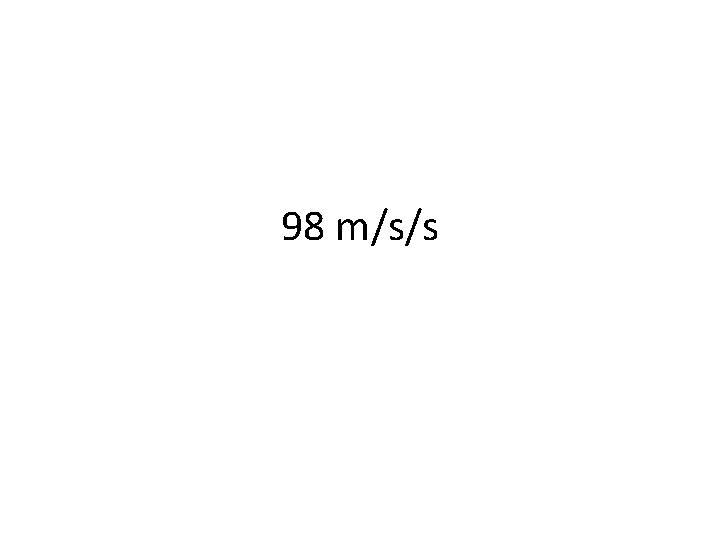98 m/s/s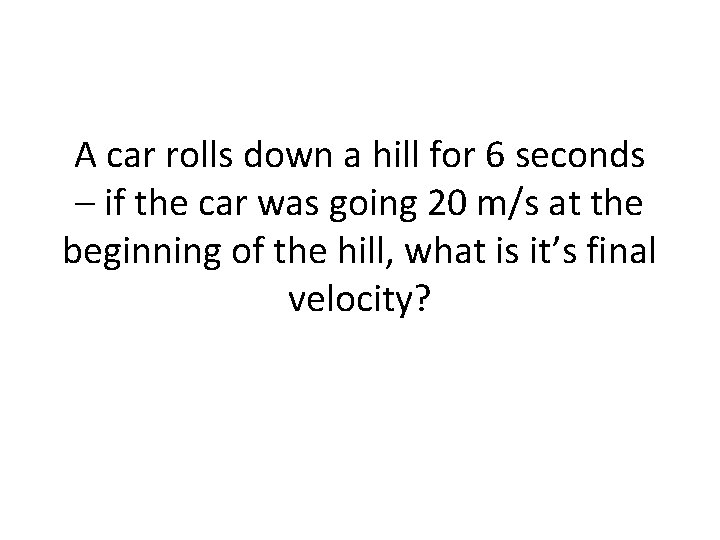A car rolls down a hill for 6 seconds – if the car was going 20 m/s at the beginning of the hill, what is it’s final velocity?78. 5 m/s